# A plane

A plane is flying at the rate of 350 mph after an eight hour flight is at its destination how far did the plane fly?

x =  2800 mi

### Step-by-step explanation: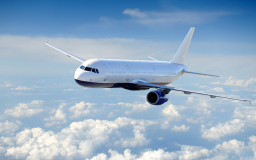Did you find an error or inaccuracy? Feel free to write us. Thank you!Tips to related online calculators
Do you want to convert length units?
Do you want to convert velocity (speed) units?
Do you want to convert time units like minutes to seconds?

## Related math problems and questions:

• Avg speed of flight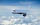The students vice adventure had a 2,367 km flight. If they travel time was 2 hours and 56 minutes, what was their average speed in kilometres per hour?
• Aircraft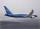If an airplane flies 846 km/h for 8 h, how far does it travel?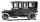Car started at 11:40 at speed 54 km/h from A and to B arrived at 12:20. Determine the distance A and B.
• Cyclist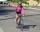Cyclist drives 5 km in half an hour, how long will drive in 3 hours?
• The swallow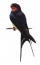The swallow will fly 2.8 km per minute. How many km will the swallow fly in one hour?
• Speed of carIn 2 hours 40 mins, a car travels 100km. At what speed is the car traveling?
• Pedestrian and cyclist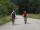Pedestrian out with a speed of 4 km/hour from city center and after 1hour and 10 minutes came after him cyclist at speed of 18 km/h. At how many minutes he catches up with pedestrian?
• Walking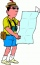The boy walked about 8.5 km in an hour. How long will it take to walk a distance of 32 km if he takes two breaks of 30 minutes during the route?
• Cyclist 9A cyclist travels at a speed of 4.25 km per hour. At that rate, how far can he travel in 3.75 hours?
• Two aircraftFrom the airport will start simultaneously two planes, which fly tracks are perpendicular to each other. The first flying speed of 680 km/h and the second 840 km/h. Calculate how far the aircraft will fly for half an hour.
• Cyclist 12What is the average speed of a cycle traveling at 20 km in 60 minutes in km/h?
• HectaresThe rectangular land is 350m long and 200m wide. Calculate the area in hectares.
• Friends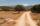Five mates traveled and the journey they lasted 195 minutes. How long would travel only one of them?
• WalkingLucie can walk about 3 4/5 miles each hour. How far can she walk in 2 hours 45 minutes?
• Aircraft bearingTwo aircraft will depart from the airport at the same time, the first with a course of 30° and the second with a course of 86°. Both fly at 330 km/h. How far apart will they be in 45 minutes of flight?
• The car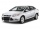The car travels at a speed of 70 km/h, what distance will it travel in 2 hours?
• Train and walker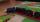Walker passes an hour 5 km. Express train per hour 100 km. Walker went 4 hours. How many minutes took the distance express train?